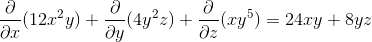# Calculus 3 : Divergence

## Example Questions

### Example Question #11 : Line Integrals

Compute the divergence of the vector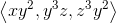.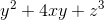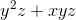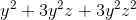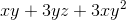Explanation:

To find the divergence of the vector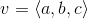,

we use the formula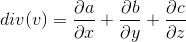.

Computing each partial derivative, we get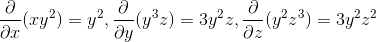.

### Example Question #12 : Line Integrals

Compute the divergence of the vector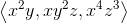.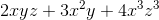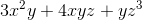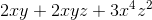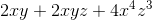Explanation:

To find the divergence of the vector, we use the formula.

Computing each partial derivative, we get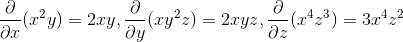.

### Example Question #13 : Line Integrals

Compute the divergence of the vector.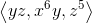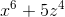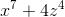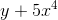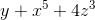Explanation:

To find the divergence of the vector, we use the formula.

Computing each partial derivative, we get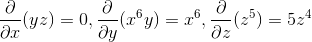.

### Example Question #14 : Line Integrals

Find the divergence of the vector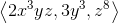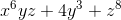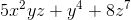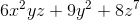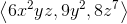Explanation:

The formula for the divergence of a vector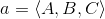is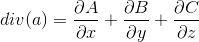. Using the vector from the problem statement, we get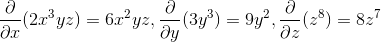. Adding them up gets us the correct answer.

### Example Question #21 : Line Integrals

Find the divergence of the vector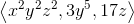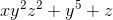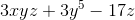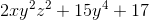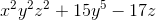Explanation:

To find the divergence a vector, you use the following definition:. Applying this to the vector from the problem statement, we get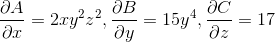. Adding all of these up, according to the definition, will produce the correct answer.

### Example Question #22 : Line Integrals

Find the divergence of the following vector: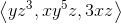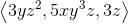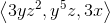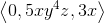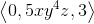Explanation:

To find the divergence a vector, you use the following definition:. Applying this to the vector from the problem statement, we get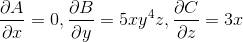. Adding all of these up, according to the definition, will produce the correct answer.

### Example Question #23 : Line Integrals

Find the divergence of the vector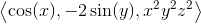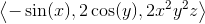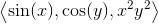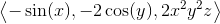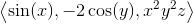Explanation:

To find the divergence a vector, you use the following definition:. Applying this to the vector from the problem statement, we get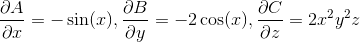. Adding all of these up, according to the definition, will produce the correct answer.

### Example Question #24 : Line Integrals

Find the divergence of the vector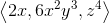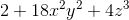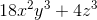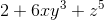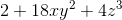Explanation:

To find the divergence of a vector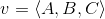, we apply the following definition: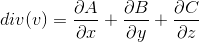. Applying the definition to the vector from the problem statement, we get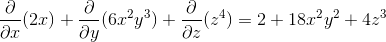### Example Question #25 : Line Integrals

Find the divergence of the vector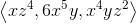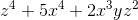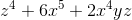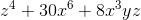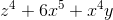Explanation:

To find the divergence of a vector, we apply the following definition:. Applying the definition to the vector from the problem statement, we get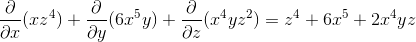### Example Question #26 : Line Integrals

Find the divergence of the vector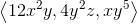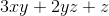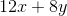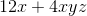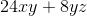To find the divergence of a vector, we apply the following definition:. Applying the definition to the vector from the problem statement, we get